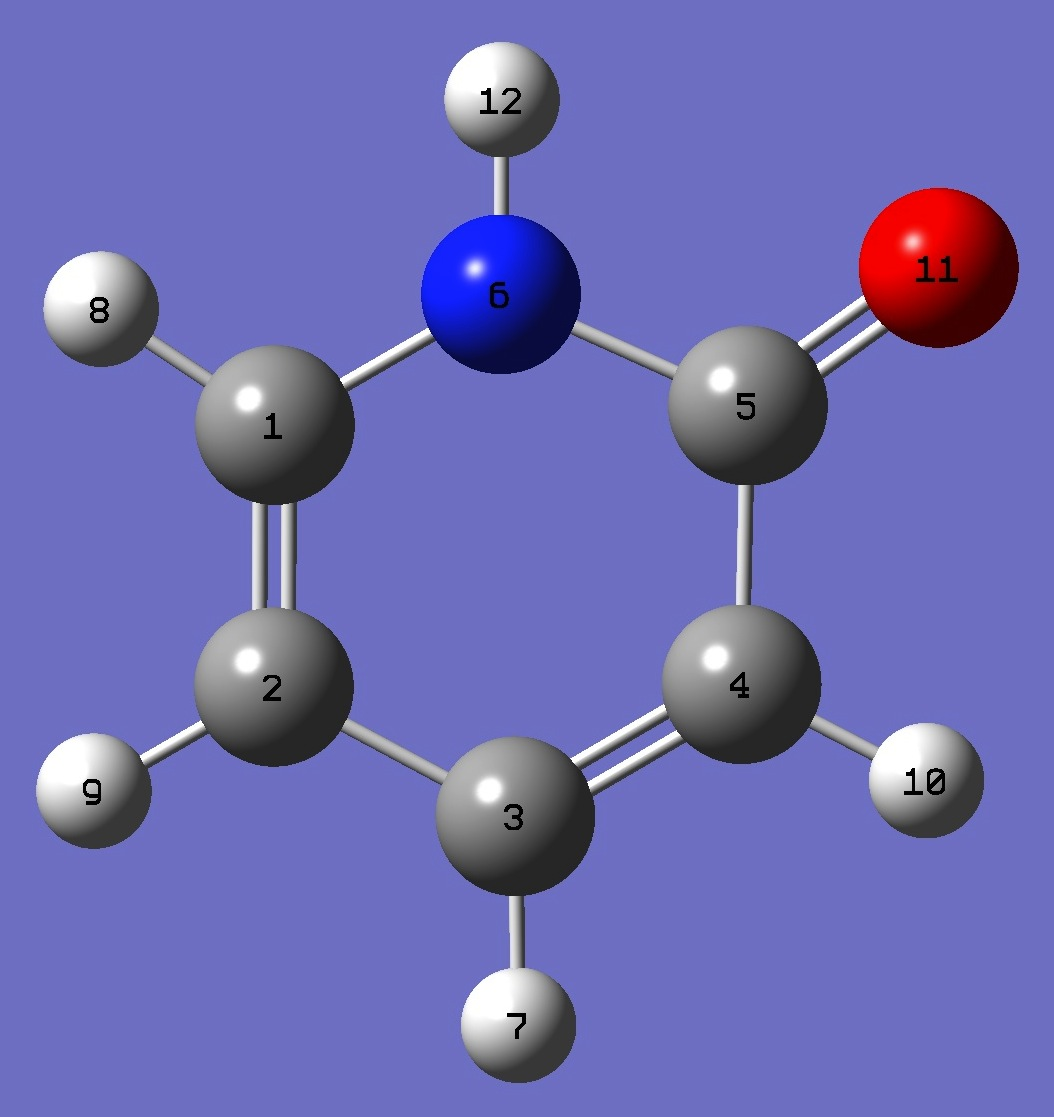2-PyridoneNitrogen and Deuterium

in 2-Pyridone

Nitrogen and Deuterium (N-D) nqcc's in 2-pyridone were determined by Tanjaroon et al. .

Calculation of the nqcc's was made here on a molecular structure derived by B3P86/6-31G(d,p) optimization.  Calculated and experimental nqcc's are compared in Tables 1 - 3.  Structure parameters are given in Table 4, rotational constants in Table 5.

In Tables 1 - 3, subscripts a,b,c refer to the principal axes of the inertia tensor; x,y,z to the principal axes of the nqcc tensor.  Ø (degrees) is the angle between its subscripted parameters.  ETA = (Xxx - Xyy)/Xzz.

RMS is the root mean square difference between calculated and experimental diagonal nqcc's (percentage of the average of the magnitudes of the experimental nqcc's).  RSD is the calibration residual standard deviation of the (1) B3PW91/6-311+G(df,pd) and (2) B3PW91/6-311+G(d,p) models for calculation of nitrogen efg's/nqcc's; and of the B3LYP/6-31G(df,3p) model for calculation of the deuterium nqcc's.

 Table 1.   14N nqcc's in 2-Pyridone (MHz).  Calculation was made on the B3P86/6-31G(d,p) optimized structure with both (1) B3PW91/6-311+G(df,pd) and (2) B3PW91/6-311+G(d,p) models. Calc. (1) Calc. (2) Expt.  Xaa 1.410 1.505 1.4962(38) Xbb 1.204 1.259 1.2691(40) Xcc - 2.615 - 2.764 - 2.7653(40) |Xab| 0.073 0.056 RMS 0.107 (5.8 %) 0.008 (0.44 %) RSD 0.030 (1.3 %) 0.086 (3.8 %) Xxx 1.181 1.247 Xyy 1.434 1.517 Xzz - 2.615 - 2.764 ETA 0.096 0.098 Øz,c 0 0

a The principal z-axis of the nqcc tensor is perpendicular to the plane of the molecule (ab-plane).

 Table 2.   14N nqcc's in 1-Deuterio-2-Pyridone (MHz).  Calculation was made on the B3P86/6-31G(d,p) optimized structure with both (1) B3PW91/6-311+G(df,pd) and (2) B3PW91/6-311+G(d,p) models. Calc. (1) Calc. (2) Expt.  Xaa 1.413 1.507 1.511(2) Xbb 1.202 1.257 1.249(5) Xcc - 2.615 - 2.764 - 2.759(5) |Xab| 0.069 0.052 RMS 0.104 (5.7 %) 0.006 (0.32 %) RSD 0.030 (1.3 %) 0.086 (3.8 %)

 Table 3.   2H nqcc's in 1-Deuterio-2-Pyridone (kHz).  Calculation was made on (1) B3P86/6-31G(d,p) and (2) B3P86/6-31G(3d,3p) optimized structures. Calc. (1) Calc. (2) Expt.  Xaa 2.1 1.6 - 110(7) Xbb 134.0 134.6 354(6) Xcc - 136.1 - 136.2 - 244(6) |Xab| 154.7 154.7 RMS 156 (66 %) 155 (66 %) RSD 1.1 (0.86 %) 1.1 (0.86 %) Xxx - 100.1 - 100.2 Xyy - 136.1 - 136.2 Xzz 236.2 236.5 ETA 0.152 0.152 Øz,a 56.54 56.64 Øa,NH 55.66 55.79 Øz,NH 0.87 0.85

 Table 4.   2-Pyridone.  B3P86/6-31G(d,p) structure parameters (Å and degrees).C  C,1,B1  C,2,B2,1,A1  C,3,B3,2,A2,1,D1,0  C,4,B4,3,A3,2,D2,0  N,1,B5,2,A4,3,D3,0  H,3,B6,2,A5,1,D4,0  H,1,B7,6,A6,5,D5,0  H,2,B8,1,A7,6,D6,0  H,4,B9,3,A8,2,D7,0  O,5,B10,4,A9,3,D8,0  H,6,B11,1,A10,2,D9,0 B1=1.36106948  B2=1.42286369  B3=1.36319773  B4=1.4483221  B5=1.35873538  B6=1.08669086  B7=1.08419652  B8=1.08195818  B9=1.08341333  B10=1.2243469  B11=1.01145096  A1=117.90194301  A2=121.49306483  A3=121.69751676  A4=120.34087458  A5=119.02079128  A6=116.24946536  A7=120.40843171  A8=122.14142012  A9=127.21849338  A10=120.42931277  D1=0.  D2=0.  D3=0.  D4=180.  D5=180.  D6=180.  D7=180.  D8=180.  D9=180. The "tautomeric" hydrogen atom is rs = 2.124(1) Å from the center of mass of the molecule .  On the B3P86/6-31G(d,p) structure, it is also 2.124 Å.

 Table 5.   2-Pyridone.  Rotational Constants (MHz).  Normal Species. Calc = B3P86/6-31G(d,p) optimization. Calc Expt.  A 5687. 5643.7580(18) B 2791. 2793.46893(71) C 1872. 1868.81993(51)

 C.Tanjaroon, R.Subramanian, C.Karunatilaka, and S.G.Kukolich, J.Phys.Chem. A 108,9531(2004).

L.D.Hatherley, R.D.Brown, P.D.Godfrey, A.P.Pierlot, W.Caminati, D.Damiani, S.Melendri, and L.B.Favero, J.Phys.Chem. 47,46(1993).

Pyridine 2-Hydroxypyridine Quinoline

Pyrazole Imidazole Isoquinoline

Molecules/Nitrogen

2Pyridone.html